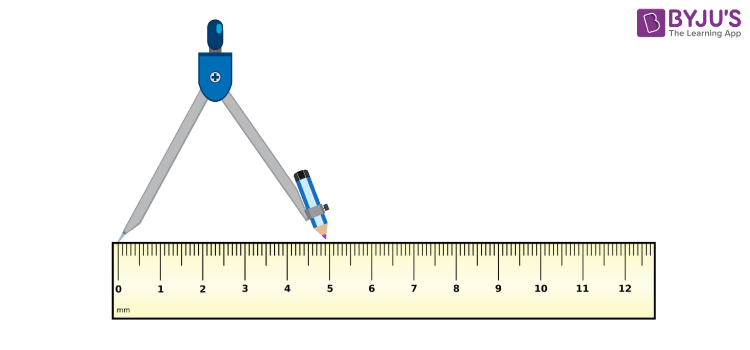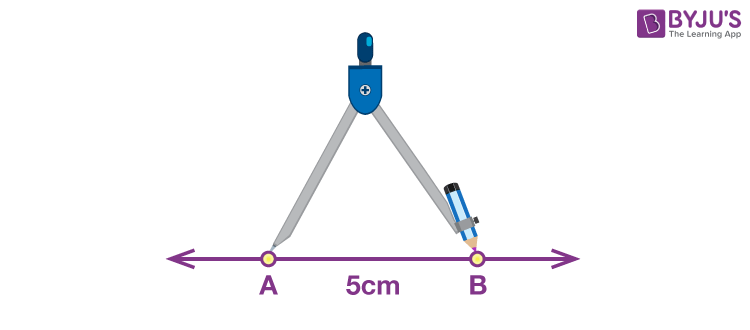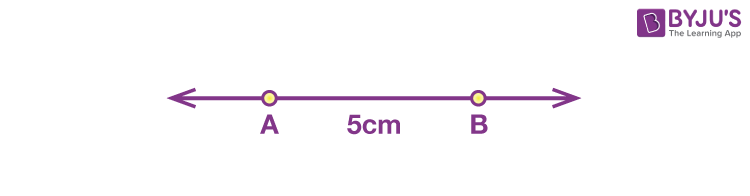Jet Set Go! All about Aeroplanes Jet Set Go! All about Aeroplanes

# Methods To Draw A Line Segment

We see a number of objects of different shapes and sizes in our day-to-day life. They help us shape and understand the basic geometrical ideas in our minds. In fact, basic geometry is nothing but the study of points, lines, line segments, angles, surfaces, and solids. Here we will focus the discussion on line segments.

A line segment is a part of a line with two endpoints. It is one of the essential elements of practical geometry as it facilitates the construction of geometric shapes and figures. This segment will enable you to learn how to draw a line segment of a given length.

The Line Segment

Before we move over to the procedure to learn how to draw a line segment, it is required for you to keep some of its characteristics in mind.

• A line segment has a distinct beginning and end.
• It is the shortest distance between two points.
• It has a fixed length.
• It is possible to measure the length of a line segment using a ruler and represent it by a diagram.

## How to draw a Line Segment of a Given Length?

To draw a line segment of a given length, we can employ either of the given methods:

• Using a ruler
• Using ruler and compasses

### Using a Ruler

We are required to draw a line segment of length 5 cm. The simplest method is by using a ruler. We mark two points using a ruler, which is at a distance of 5 cm from each other. Then, mark the points as A and B and join them. We get a line segment AB of length 5 cm

However, it is to be noted that if we do not look straight down at the ruler, we might end up capturing an incorrect distance. A more accurate method for the same is described below.

### Using Ruler and Compasses

The steps to draw a line segment of length 5 cm using a ruler and compasses are:

• Step 1: Draw a line of any length. Mark a point A on the line, which is the starting point of the line segment.
• Step 2: Using a ruler, place the pointer of the compass 5 cm apart from the pencil’s lead.• Step 3: Place the pointer of the compass at A and mark an arc on the line with the pencil point.• Step 4: Mark the point where the arc and the line intersect at B.
• Step 5: AB is the required line segment of length 5 cm.## Frequently Asked Questions – FAQs

### How many endpoints does a line segment have?

There are two endpoints in a line segment.

### How to draw a line segment of a given length?

Using a ruler and a compass we can draw a line segment.

### If a line has only one endpoint then it is called?

If a line has only one endpoint then it is called a ray.

### Define line segment.

Line segment is a part of a line which has two endpoints.

### Write the examples of figures which we can draw using line segment.

Using line segments we can draw triangles, rectangles and squares etc.

To know more about different methods to draw a line segment or other geometrical figures, download BYJU’S The Learning App.

Test your Knowledge on Methods Draw Line Segment